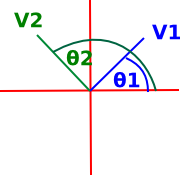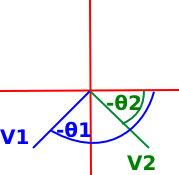# Maths - Issues with Relative Angles

There are some issues with taking the relative angle between two angles, especially if we use this formula:
atan2(v2.y,v2.x) - atan2(v1.y,v1.x)

It is useful to keep in mind that:

• We can always add or subtract 360° (or in radians 2π) to any angle without changing the angle it represents (because after 360° we get back to where we started ). In most implementations atan2 will return a value between +π and -π but if we want a value between 0 and 2π then we could add 2π to negative values.
• If atan2 returns a value between +π and -π then
atan2(v2.y,v2.x) - atan2(v1.y,v1.x)
could return a value between +2π and -2π so if it is above +π we might want to subtract 2π and if it is below -π we might want to add 2π.
• If we are facing in direction 1 and we want to rotate to face in direction 2 we could rotate clockwise or anti-clockwise. So a rotation of pi/2 in the clockwise direction is the same as in the anti-clockwise direction 3pi/4. So we need to be clear if we always want to rotate in a clockwise direction or if we want to rotate clockwise or anti-clockwise depending on which is the smaller angle.

So, as an example, lets look at two vectors which are 90° (π/2) apart relative to each other. It should not matter what coordinate system we use as follows:If v1 is in the first quadrant and v2 is in the second we might get: atan2(-1,1) - atan2(1,1) = 3/4π - 1/4π = π/2If v1 is in the second quadrant and v2 is in the third we might get: atan2(-1,-1) - atan2(-1,1) = -3/4π - 3/4π = -π3/2 adding 2π gives: = π/2If v1 is in the third quadrant and v2 is in the forth we might get: atan2(1,-1) - atan2(-1,-1) = -1/4π + 3/4π = π/2If v1 is in the forth quadrant and v2 is in the first we might get: atan2(1,1) - atan2(1,-1) = 1/4π + 1/4π = π/2

So the angle between the vectors is always π/2.

 metadata block see also: Correspondence about this page This page arose from a forum discussion with Jason about calculating relative angles here. Book Shop - Further reading. Where I can, I have put links to Amazon for books that are relevant to the subject, click on the appropriate country flag to get more details of the book or to buy it from them.If you are interested in 3D games, this looks like a good book to have on the shelf. If, like me, you want to have know the theory and how it is derived then there is a lot for you here. Including - Graphics pipeline, scenegraph, picking, collision detection, bezier curves, surfaces, key frame animation, level of detail, terrain, quadtrees & octtrees, special effects, numerical methods. Includes CDROM with code. Other Math Books Specific to this page here:

This site may have errors. Don't use for critical systems.